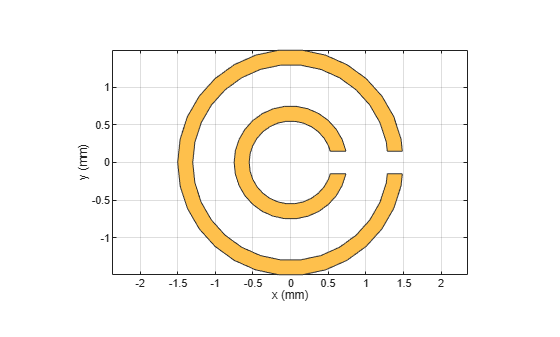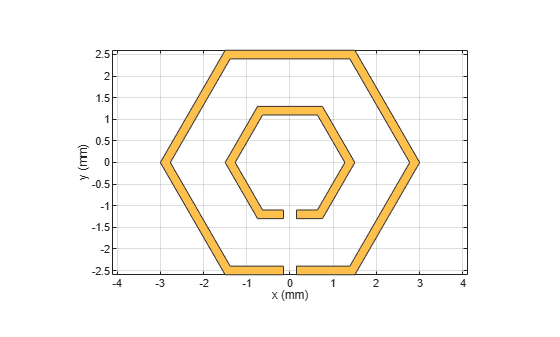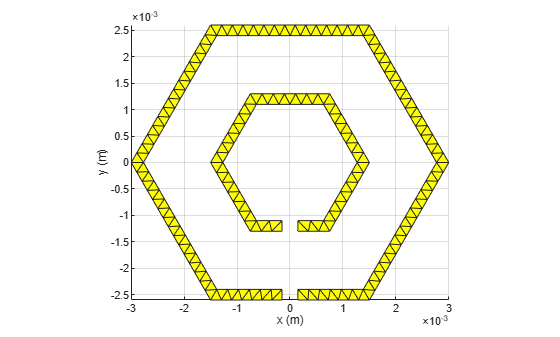# splitRing

Create split ring shape on X-Y plane

Since R2023a

## Description

Use the `splitRing` object to create a split-ring shape centered at the origin on the X-Y plane.

## Creation

### Syntax

``splitringshape = splitRing``
``splitringshape = splitRing(Name=Value)``

### Description

example

````splitringshape = splitRing` creates a split-ring shape centered at the origin and on the X-Y plane.```

example

````splitringshape = splitRing(Name=Value)` sets Properties using one or more name-value arguments. For example, `splitRing(ReferencePoint=[1 1])` creates a split ring shape at the reference point `[1 1]`. Properties not specified retain their default values.```

## Properties

expand all

Name of the split-ring shape, specified as a character vector or string scalar.

Example: `splitringshape = splitRing(Name='splitringshape1')`

Data Types: `char` | `string`

Shape of split ring, specified as: `'Circle'`, `'Triangle'`, `'Square'`, `'Hexagon'`, or `'Octagon'`.

Example: `splitringshape = splitRing(Type='Hexagon')`

Data Types: `char`

Reference point of the split-ring shape in Cartesian coordinates, specified as a two-element vector.

Example: `splitringshape = splitRing(ReferencePoint=[1 1])`

Data Types: `double`

Diameter of the inner ring in meters, specified as a positive scalar.

Example: `splitringshape = splitRing(RingDiameterInner=0.0025)`

#### Dependencies

To enable this property, set `Type` to `'Circle'`.

Data Types: `double`

Diameter of the outer ring in meters, specified as a positive scalar.

Example: `splitringshape = splitRing(RingDiameterOuter=0.0040)`

#### Dependencies

To enable this property, set `Type` to `'Circle'`.

Data Types: `double`

Side length of the inner ring in meters, specified as a positive scalar.

Example: `splitringshape = splitRing(SideLengthInner=0.0025)`

#### Dependencies

To enable this property, set `Type` to `'Triangle'`, `'Hexagon'`, `'Square'`, or `'Octagon'`.

Data Types: `double`

Side length of the outer ring in meters, specified as a positive scalar.

Example: `splitringshape = splitRing(SideLengthOuter=0.0030)`

#### Dependencies

To enable this property, set `Type` to `'Triangle'`, `'Hexagon'`, `'Square'`, or `'Octagon'`.

Data Types: `double`

Width of the inner and the outer rings in meters, specified as a positive scalar.

Example: `splitringshape = splitRing(TraceWidth=5.0000e-04)`

Data Types: `double`

Angle of the split between the inner and outer ring in degrees, specified as a two-element vector.

Example: `splitringshape = splitRing(SplitAngle=[10 10])`

#### Dependencies

To enable this property, set `Type` to `'Circle'`.

Data Types: `double`

Side of the split between the inner and outer ring in meters, specified as a two-element vector.

Example: `splitringshape = splitRing(SplitSide=[2 2])`

#### Dependencies

To enable this property, set `Type` to `'Triangle'`, `'Hexagon'`, `'Square'`, or `'Octagon'`.

Data Types: `double`

Width of split on both inner and outer ring in meters, specified as a positive scalar.

Example: `splitringshape = splitRing(SplitSide=2.0000e-04)`

#### Dependencies

To enable this property, set `Type` to `'Triangle'`, `'Hexagon'`, `'Square'`, or `'Octagon'`.

Data Types: `double`

## Object Functions

 `add` Boolean unite operation on two RF PCB shapes `and` Shape1 & Shape2 for RF PCB shapes `area` Calculate area of RF PCB shape in square meters `intersect` Boolean intersection operation on two RF PCB shapes `mesh` Change and view mesh properties of metal or dielectric in PCB component `minus` Shape1 - Shape2 for RF PCB shapes `plus` Shape1 + Shape2 for RF PCB shapes `rotate` Rotate RF PCB shape about defined axis `rotateX` Rotate RF PCB shape about x-axis `rotateY` Rotate RF PCB shape about y-axis and angle `rotateZ` Rotate RF PCB shape about z-axis `subtract` Boolean subtraction operation on two RF PCB shapes `scale` Change size of RF PCB shape by fixed amount `show` Display PCB component structure or PCB shape `translate` Move RF PCB shape to new location

## Examples

collapse all

Create a default split-ring shape.

`splitringshape = splitRing`
```splitringshape = splitRing with properties: Name: 'mysplitring' Type: 'Circle' ReferencePoint: [0 0] RingDiameterInner: 0.0015 RingDiameterOuter: 0.0030 TraceWidth: 2.0000e-04 SplitGap: 3.0000e-04 SplitAngle: [0 0] ```

View the shape.

`show(splitringshape)`Create a hexagonal split ring.

```splitringshape = splitRing('Type','Hexagon'); show(splitringshape)```Mesh it with maximum edge length of 0.5 mm.

`figure; mesh(splitringshape,'MaxEdgeLength',0.5e-3);`## Version History

Introduced in R2023a